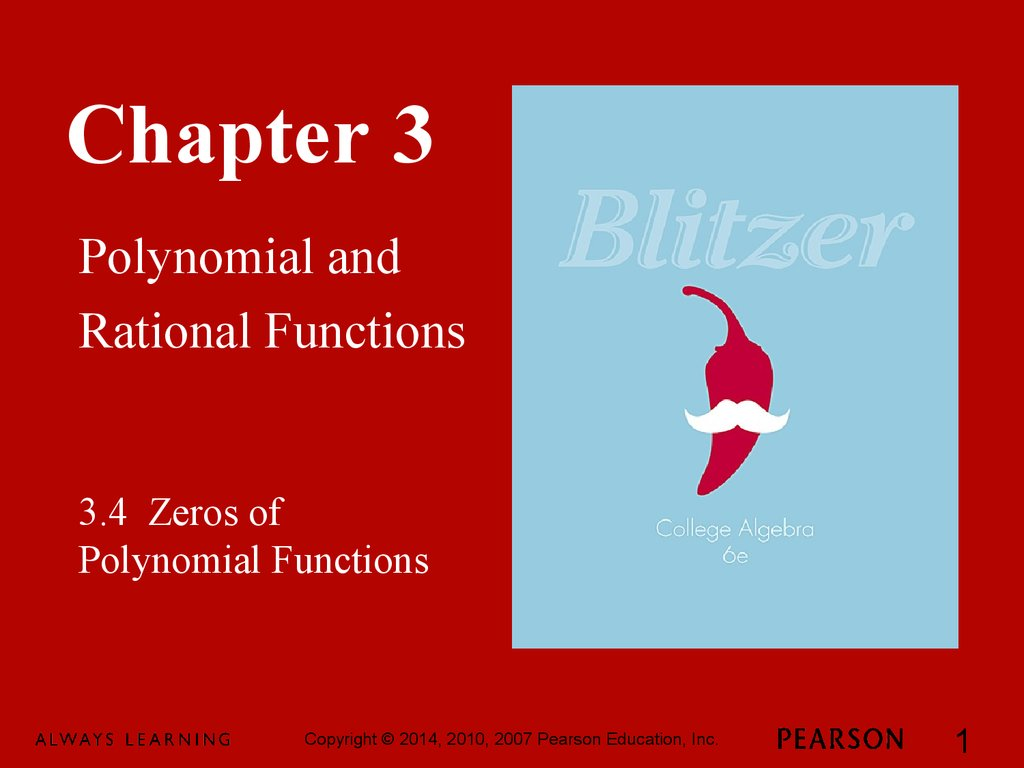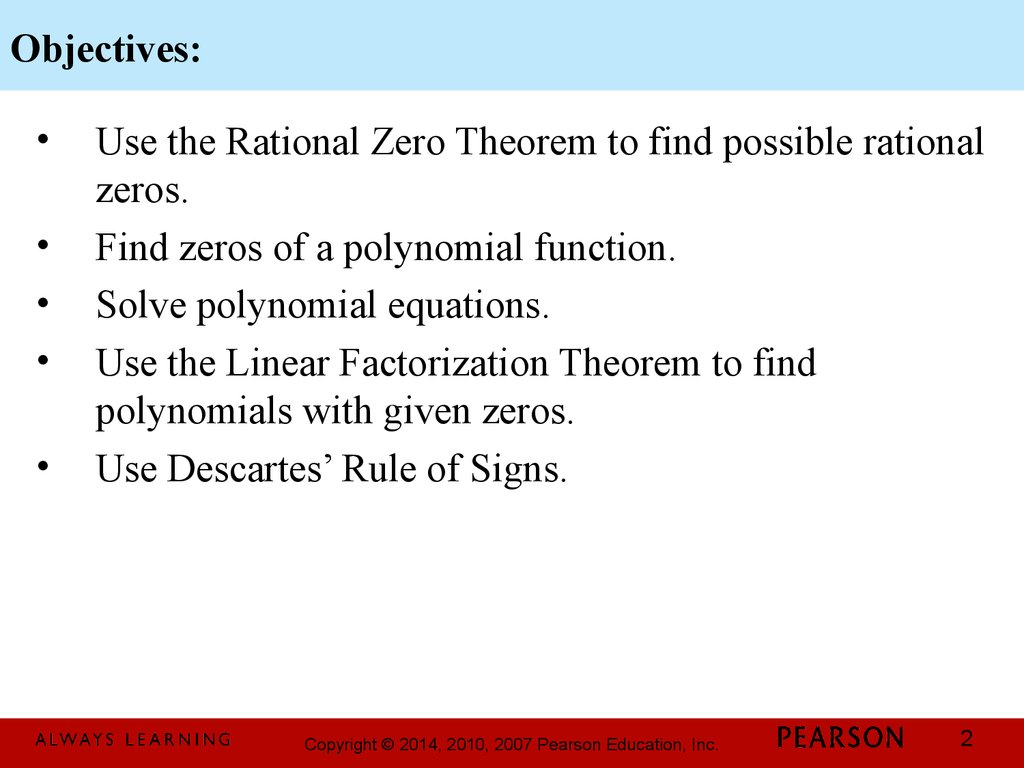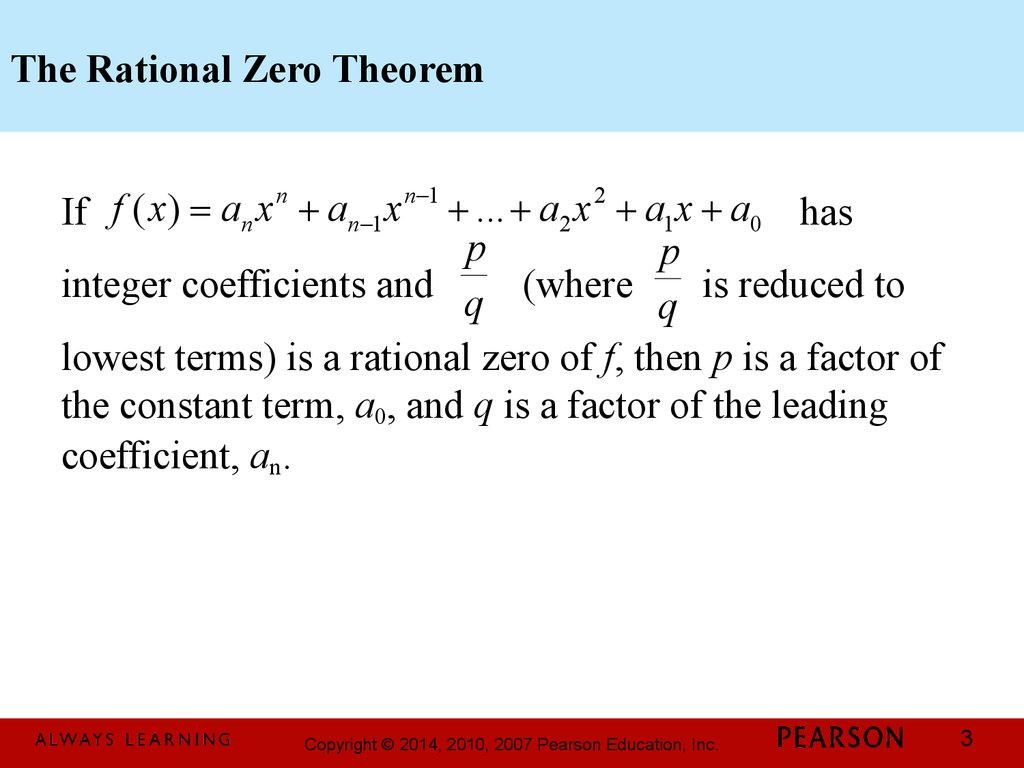# Chapter 3. Polynomial and Rational Functions. 3.4 Zeros of Polynomial Functions

## 1.

Chapter 3
Polynomial and
Rational Functions
3.4 Zeros of
Polynomial Functions
1

## 2. Objectives:

Use the Rational Zero Theorem to find possible rational
zeros.
Find zeros of a polynomial function.
Solve polynomial equations.
Use the Linear Factorization Theorem to find
polynomials with given zeros.
Use Descartes’ Rule of Signs.
2

## 3. The Rational Zero Theorem

n
n -1
2
f
(
x
)
=
a
x
+
a
x
+
...
+
a
x
+ a1x + a0 has
If
n
n -1
2
p
p
integer coefficients and q (where
is reduced to
q
lowest terms) is a rational zero of f, then p is a factor of
the constant term, a0, and q is a factor of the leading
coefficient, an.
3

## 4. Example: Using the Rational Zero Theorem

List all possible rational zeros of f ( x) = 4 x 5 + 12 x 4 - x - 3
The constant term is –3 and the leading coefficient is 4.
Factors of the constant term, –3: ±1, ±3
Factors of the leading coefficient, 4: ±1, ±2, ±4
factors of - 3
±1, ±3
=
factors of 4
±1, ±2, ±4
Possible rational zeros are: ±1, ±3, ± 1 , ± 1 , ± 3 , ± 3
2 4 2 4
4

## 5. Example: Finding Zeros of a Polynomial Function

Find all zeros of f ( x) = x + x - 5 x - 2
3
2
We begin by listing all possible rational zeros.
±1, ±2
= ±1, ±2
Possible rational zeros =
±1
We now use synthetic division to see if we can find a
rational zero among the four possible rational zeros.
5

## 6. Example: Finding Zeros of a Polynomial Function (continued)

Find all zeros of f ( x) = x + x - 5 x - 2
3
2
Possible rational zeros are 1, –1, 2, and –2. We will use
synthetic division to test the possible rational zeros.
-2 + 1 +1 -5 -2
-2 +2 +6
1 -1 -3 +4
-1 + 1 +1 -5 -2
-1 0 5
1 0 -5 3
Neither –2 nor –1 is a zero. We continue testing
possible rational zeros.
6

## 7. Example: Finding Zeros of a Polynomial Function (continued)

Find all zeros of f ( x) = x + x - 5 x - 2
Possible rational zeros are 1, –1, 2, and –2. We will use
synthetic division to test the possible rational zeros. We
have found that –2 and –1 are not rational zeros. We
continue testing with 1 and 2.
1 + 1 +1 -5 -2
2 + 1 +1 -5 -2
1 2 -3
2 +6 +2
1 2 -3 -5
1 +3 +1 0
3
2
We have found a rational zero at x = 2.
7

## 8. Example: Finding Zeros of a Polynomial Function (continued)

Find all zeros of f ( x) = x + x - 5 x - 2
We have found a rational zero at x = 2.
The result of synthetic division is:
2 + 1 +1 -5 -2
2 +6 +2
1 +3 +1 0
3
2
3
2
2
x
+
x
5
x
2
=
(
x
2)(
x
+ 3 x + 1).
This means that
2
x
+ 3 x + 1 = 0.
We now solve
8

## 9. Example: Finding Zeros of a Polynomial Function (continued)

3
2
f
(
x
)
=
x
+
x
- 5x - 2
Find all zeros of
3
2
2
x
+
x
5
x
2
=
(
x
2)(
x
+ 3 x + 1).
We have found that
We now solve x 2 + 3 x + 1 = 0.
-3 ± 5
-b ± b 2 - 4ac -3 ± 32 - 4(1)(1)
=
x=
=
2
2a
2(1)
The solution set is
ì -3 + 5 -3 - 5 ü
,
í2,
ý.
2
2 þ
î
The zeros of
3
2
f ( x) = x + x - 5 x - 2
are
-3 + 5
-3 - 5
2,
, and
2
2
9

## 10. Properties of Roots of Polynomial Equations

1. If a polynomial equation is of degree n, then
counting multiple roots separately, the equation has n
roots.
2. If a + bi is a root of a polynomial equation with real
coefficients (b ¹ 0), then the imaginary number
a – bi is also a root. Imaginary roots, if they exist,
occur in conjugate pairs.
10

## 11. Example: Solving a Polynomial Equation

Solve x 4 - 6 x 3 + 22 x 2 - 30 x + 13 = 0
We begin by listing all possible rational roots:
±1, ±13
Possible rational roots =
±1
Possible rational roots are 1, –1, 13, and –13. We will
use synthetic division to test the possible rational zeros.
11

## 12. Example: Solving a Polynomial Equation (continued)

Solve x 4 - 6 x 3 + 22 x 2 - 30 x + 13 = 0
Possible rational roots are 1, –1, 13, and –13. We will use
synthetic division to test the possible rational zeros.
-1 +1 -6 +22 -30 +13
-1 7 -29 +59
1 -7 +29 -59 +72
1 +1 -6 +22 -30 +13
+1 -5 +17 -13
1 -5 +17 -13 0
x = 1 is a root for this polynomial.
We can rewrite the equation in factored form
4
3
2
3
2
x - 6 x + 22 x - 30 x + 13 = ( x - 1)( x - 5 x + 17 x - 13)
12

## 13. Example: Solving a Polynomial Equation (continued)

Solve x 4 - 6 x 3 + 22 x 2 - 30 x + 13 = 0
We have found that x = 1 is a root for this polynomial.
In factored form, the polynomial is
x 4 - 6 x 3 + 22 x 2 - 30 x + 13 = ( x - 1)( x 3 - 5 x 2 + 17 x - 13)
We now solve x 3 - 5 x 2 + 17 x - 13 = 0
We begin by listing all possible rational roots.
Possible rational roots = ±1, ±13
±1
Possible rational roots are 1, –1, 13, and –13. We will
use synthetic division to test the possible rational zeros.
13

## 14. Example: Solving a Polynomial Equation (continued)

Solve x 4 - 6 x 3 + 22 x 2 - 30 x + 13 = 0
Possible rational roots are 1, –1, 13, and –13. We will
use synthetic division to test the possible rational zeros.
Because –1 did not work for the original polynomial, it
is not necessary to test that value.
1 +1 -5 +17 -13
x = 1 is a (repeated) root
1 -4 +13
for this polynomial
1 -4 +13 0
The factored form of this polynomial is
4
3
2
2
x - 6 x + 22 x - 30 x + 13 = ( x - 1)( x - 1)( x - 4 x + 13)
14

## 15. Example: Solving a Polynomial Equation (continued)

Solve x 4 - 6 x 3 + 22 x 2 - 30 x + 13 = 0
The factored form of this polynomial is
x 4 - 6 x 3 + 22 x 2 - 30 x + 13 = ( x - 1)( x - 1)( x 2 - 4 x + 13)
x -1 = 0 ® x = 1
2
x
- 4 x + 13 = 0
We will use the quadratic formula to solve
-b ± b - 4ac 4 ± (-4) - 4(1)(13)
4 ± -36
x=
=
=
2a
2(1)
2
2
2
4 ± 6i
=
= 2 ± 3i
2
The solution set of the original
equation is { 1,1,2 ± 3i}
15

## 16. The Fundamental Theorem of Algebra

If f(x) is a polynomial of degree n, where n ³ 1, then the
equation f(x) = 0 has at least one complex root.
16

## 17. The Linear Factorization Theorem

n -1
If f ( x) = an x + an-1 x + ... + a2 x + a1x + a0 , where
n ³ 1 and an ¹ 0, then
f ( x) = an ( x - c1 )( x - c2 )...( x - cn )
n
2
where c1, c2, ..., cn are complex numbers (possibly real
and not necessarily distinct). In words: An nth-degree
polynomial can be expressed as the product of a
nonzero constant and n linear factors, where each linear
factor has a leading coefficient of 1.
17

## 18. Example: Finding a Polynomial Function with Given Zeros

Find a third-degree polynomial function f(x) with real
coefficients that has –3 and i as zeros and such that
f(1) = 8.
Because i is a zero and the polynomial has real
coefficients, the conjugate, –i, must also be a zero. We
can now use the Linear Factorization Theorem.
f ( x) = an ( x - c1 )( x - c2 )...( x - cn )
f ( x) = an ( x + 3)( x - i )( x + i ) = an ( x + 3)( x 2 + 1)
f ( x) = an ( x 3 + 3 x 2 + x + 3)
18

## 19. Example: Finding a Polynomial Function with Given Zeros

Find a third-degree polynomial function f(x) with real
coefficients that has –3 and i as zeros and such that
f(1) = 8.
Applying the Linear Factorization Theorem, we found
3
2
f
(
x
)
=
a
(
x
+
3
x
+ x + 3).
that
n
f (1) = an (13 + 3g12 + 1 + 3) = 8
an (1 + 3 + 1 + 3) = 8
The polynomial function is
8an = 8
f ( x) = x3 + 3x 2 + x + 3
an = 1
19

## 20. Descartes’ Rule of Signs

Let f ( x) = an x n + an-1 x n-1 + ... + a2 x 2 + a1x + a0
be a polynomial with real coefficients.
1. The number of positive real zeros of f is either
a. the same as the number of sign changes of f(x)
or
b. less than the number of sign changes of f(x) by a
positive even integer. If f(x) has only one
variation in sign, then f has exactly one positive
real zero.
20

## 21. Descartes’ Rule of Signs (continued)

Let f ( x) = an x n + an-1 x n-1 + ... + a2 x 2 + a1 x + a0
be a polynomial with real coefficients.
2. The number of negative real zeros of f is either
a. The same as the number of sign changes in f(–x)
or
b. less than the number of sign changes in f(–x) by
a positive even integer. If f(–x) has only one
variation in sign, then f has exactly one negative
real zero
21

## 22. Example: Using Descartes’ Rule of Signs

Determine the possible number of positive and negative
4
3
2
real zeros of f ( x) = x - 14 x + 71x - 154 x + 120
1. To find possibilities for positive real zeros, count the
number of sign changes in the equation for f(x).
There are 4 variations in sign.
The number of positive real zeros of f is either 4, 2, or 0.
22

## 23. Example: Using Descartes’ Rule of Signs

Determine the possible number of positive and negative
4
3
2
real zeros of f ( x) = x - 14 x + 71x - 154 x + 120
2. To find possibilities for negative real zeros, count the
number of sign changes in the equation for f(–x).
f (- x) = (- x) 4 - 14(- x)3 + 71(- x) 2 - 154( - x) + 120
f (- x) = x 4 + 14 x 3 + 71x 2 + 154 x + 120
There are no variations in sign.
There are no negative real roots for f.